Courses

# Single Component System Phase Equilibria Civil Engineering (CE) Notes | EduRev

## Civil Engineering (CE) : Single Component System Phase Equilibria Civil Engineering (CE) Notes | EduRev

The document Single Component System Phase Equilibria Civil Engineering (CE) Notes | EduRev is a part of the Civil Engineering (CE) Course Thermodynamics.
All you need of Civil Engineering (CE) at this link: Civil Engineering (CE)

Single Component System Phase Equilibria

We start with the general criterion of equality of the chemical potential in the two phases. To generalize the results we assume that any two types of phases α and β of a pure component are at equilibrium. Thus as given by eqn. 6.50: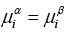...(6.50)

However, for a pure component the chemical potential is reduces to the pure component molar Gibbs free energy. Therefore: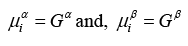...(7.1)
Thus eqn. 6.50 reduces to: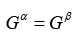...(7.2)

On taking a differential: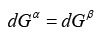...(7.3)

Using the generic relationship in eqn. 5.7 we may write in keeping with the fact that for a given equilibrium temperature, the equilibrium pressure corresponds to the saturation vapour pressure Psat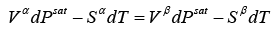...(7.4)
On rearranging: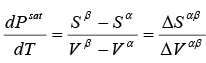...(7.5)

Additionally using the second law we have: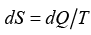...(7.6)
And that for a constant pressure process: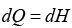...(7.7)

Using eqns. (7.6) and (7.7) we obtain:

∆Hαβ  = T ∆Sαβ                              ...(7.8)
Thus, ∆Sαβ= ∆Hαβ / T , and substitution in eqn.7.5 gives: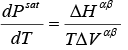...(7.9)

The last equation is called the Clapeyron equation. For the specific case of phase transition from liquid (l) to vapor (v), it translates into: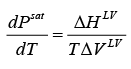...(7.10)

Noting that liquid phase molar volumes are relatively much lesser than vapour phase volumes, we may write,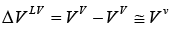...(7.11)

Further at low to moderate saturation pressures if we assume ideal vapour phase behaviour, then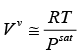...(7.12)

Eqn. 7.10 then becomes: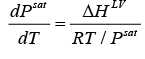Or: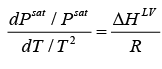...(7.13)

Whence,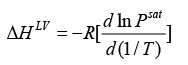...(7.14)

This approximate equation is known as the Clausius-Clapeyron equation. The assumptions used in the above derivations have approximate validity only at low pressures. Integrating eqn. 7.14 we have:

ln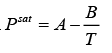...(7.15)

On comparing eqns. 7.14 and 7.15, it follows that: B = ∆HLV / R, while A is the constant of integration. These are generally regarded as constants for a given species. A plot of experimental values of lnPsat vs. 1/T generally yields a line that is nearly straight between the triple and critical points. However, the validity of eqn. 7.15 is questionable at relatively high pressures, and certainly in the critical region.Thus the accuracy of the Clausius-Clapeyron equation reduces at higher pressures. A modified form of eqn. 7.15, called the Antoine Equation, has proved to be more accurate (including at higher pressures), has the following form:

In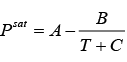...(7.16)

A, B, and C are readily available for a large number of species. Appendix VI provides values of Antoine constants for select substances. More complex forms of equations relation temperature and vapour pressure of pure substances have been reported in the literature, which provide even greater accuracy. An example of such an equation is the Wagner equation, which is given by:

In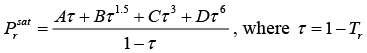...(7.17)

Offer running on EduRev: Apply code STAYHOME200 to get INR 200 off on our premium plan EduRev Infinity!

## Thermodynamics

56 videos|92 docs|33 tests

,

,

,

,

,

,

,

,

,

,

,

,

,

,

,

,

,

,

,

,

,

;